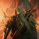1281 obejrzeń
1281
A strategy for the Dax using ema stochastic and trail_points =40
```//@version=2
strategy("DaxK_Limit", overlay=true)
length = input(14, minval=1)
OverBought = input(52)
OverSold = input(48)
smoothK = 1
smoothD = 3

k = ema(stoch(close, high, low, length), smoothK)
d = ema(k, smoothD)

if (not na(k) and not na(d))
if (crossover(k,d) and k < OverSold)
strategy.entry("StochLE", strategy.long)
strategy.exit("StochLE", trail_points=40)
if (crossunder(k,d) and k > OverBought)
strategy.entry("StochSE", strategy.short)
strategy.exit("StochSE",trail_points=40)

//plot(strategy.equity, title="equity", color=red, linewidth=2, style=areabr)```cjszbd
Repaint how? Do you mean incorporate new data as time goes on? I'm sorry I'm new to pine script. How do I do that?
OdpowiedźEntity
I don't think it repaints and it works on normal candles as well
Odpowiedź